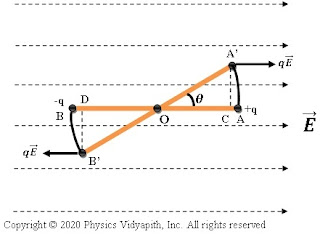### The electric potential energy of an electric dipole in the uniform electric field

Derivation of the electric potential energy of an electric dipole in the uniform electric field:

Let us consider an electric dipole $AB$, which is made up of two charges $q_1$ and $q_2$, which are placed at a distance of $2l$ in the electric field $E$. So force acting on each charge due to the electric field will be $qE$. If the dipole gets rotated a small-angle $d\theta$ against the torque acting on it in the uniform electric field $E$ then the small work done is

$dW=\tau. d\theta \qquad (1)$Force of moment on an electric Dipole
The torque (i.e moment of force) on an electric dipole in a uniform electric field

$\tau=p.E\:sin\theta$

Now substitute the value of $\tau$ in equation $(1)$. So work done

$dW=p.E\:sin\theta.d\theta$

If the dipole rotate the angle from $\theta_{1}$ to angle $\theta_{2}$ then workdone

$\int_{W_{1}}^{W_{2}}dW=p.E\int_{\theta_{1}}^{\theta_{2}}sin\theta \: d\theta$

$W_{2}-W_{1}=p.E\left[-cos\theta \right]_{\theta_{1}}^{\theta_{2}}$

$\Delta W= p.E \left( cos\theta_{1}-cos\theta_{2} \right)\qquad\qquad (2)$

This work is stored in the form of the electric potential energy of an electric dipole in the electric field. So

$U=\Delta W$

$U=p.E \left( cos\theta_{1}-cos\theta_{2} \right)$

If the electric dipole rotates from $0^{\circ}$ (when the direction of electric dipole moment $p$ is aligned in the direction of the electric field $E$) to an angle $\theta$ in the electric field i.e $\theta_{1}=0^{\circ}$ and $\theta_{2}=\theta$ then the electric potential energy of dipole in a uniform electric field

$U=p.E (1-cos\theta)$

Case-(I) If $\theta=0^{\circ}$ i.e It is stable equilibrium position then

$U_{min}=-pE$

Case-(II) If $\theta=90^{\circ}$ i.e Position of zero energy then

$U=0$

Case-(III) If $\theta=180^{\circ}$ i.e It is unstable equilibrium position then

$U_{max}=pE$